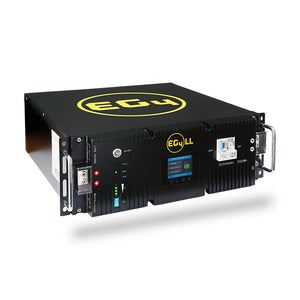Starter Kits [HERE]# Battery(s) & Generator(s)

### Which Battery is right for me?

When sizing your system its important to have a good understanding of your usage. Energy In -->[Battery]--> Energy Out. Our most important tools for this, is our Voltmeter and the Equation; Volts x Amps = Watts.

WATTS (W) = IN THE MOMENT ENERGY USEAGE

If someone were to measure their continuous energy usage (WATTS) at idle, aka going about a normal day, then one can determine what would be considered the minimum Solar Array or "Photovoltaic System" input is needed.

Ex. 1) I have (3) 100 Watt Solar Panels coming in at 300 Watts  collectively and I'm using(creating a "LOAD" or "DRAW") of 200 Watts, then my battery(s) will stay "FULL".

Ex. 2) I have (3) 100 Watt Solar Panels coming in at 300 Watts and I'm using (creating a "LOAD" or "DRAW") 400 Watts my battery(s) will slowly "DRAIN".

WATT HOURS (Wh) = ENERGY OVER TIME

If someone were to measure their energy over time or "WATT HOURS" (Wh) though out a 24 hour period, then one can determine what would be considered an adequate amount of "Sun Hours", aka hours of direct sun, needed per day to fill a battery to its max "CAPASITY" also measured in Wh.

Ex. 1) I have a battery that measures 12 Volts and 100 Amp Hours  giving the battey a known "Capacity" of 1200 Watt Hours.

EX. 2) I have a 1200 Wh battery and I want to charge it off my (2) 100 W Solar Panels. With some simple math we can see that 1200Wh / 200W = 6 Hours of required "Sun Hours". I can run this example backwards to determine my outgoing energy.

Below is an example of a common Wiring Diagram of small scale, off grid, solar systems to use as a base reference.**Remember, most of the time the best Solar Panel(s) for the job is whatever you have or can get.""